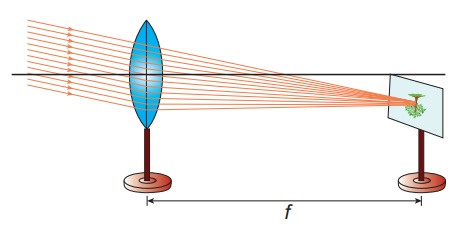Home | | Science 10th Std | Determination of focal length of a convex lens

# Determination of focal length of a convex lens

To determine the focal length of a convex lens by using 1. Distant object method 2. uv method

DETERMINATION OF FOCAL LENGTH OF A CONVEX LENS

### Aim:

To determine the focal length of a convex lens by using

1. Distant object method

2. uv method

### Apparatus required:

A convex lens, stand, wire gauze object, screen and measuring scale.

### Formula:Here,

u is the distance between the object (light source) and the convex lens

v is the distance of the image (screen) from the convex lens

f is the focal length of the convex lens

### 1. Distant Object Method:

Fix the given convex lens vertically on the stand and place it on the table near an open window of the laboratory. Locate a distant object (tree or building) through the open window. Place the screen behind the convex lens. Adjust the position of the convex lens and the screen so as to get a sharp, inverted and diminished image. Measure the distance between the screen and the convex lens with the help of the measuring scale. This distance is equal to the approximate focal length of the convex lens (f)### 2. uv - Method:

Fix the given convex lens vertically on the stand and place it on the table. Place the wire gauze object on the left side of the convex lens (say at a distance greater than 2f). Measure the distance between the object and the lens (u). Place the screen on the right side of the convex lens and adjust its position to get a sharp, inverted and diminished image. Measure the distance between the screen and the lens (v). Repeat the same procedure, by changing the distance of the object (u) and tabulate your observations.### Observation:

Focal length of the convex lens (By distance object method) is (f) = …………cm

2f = …….cm### Result:

The focal length of the given convex lens

1. By distance object method f = ………cm

2. By ‘uv’ method f = … .…..cm

Tags : Physics Laboratory Practical Experiment , 10th Science : Physics Practicals
Study Material, Lecturing Notes, Assignment, Reference, Wiki description explanation, brief detail
10th Science : Physics Practicals : Determination of focal length of a convex lens | Physics Laboratory Practical Experiment